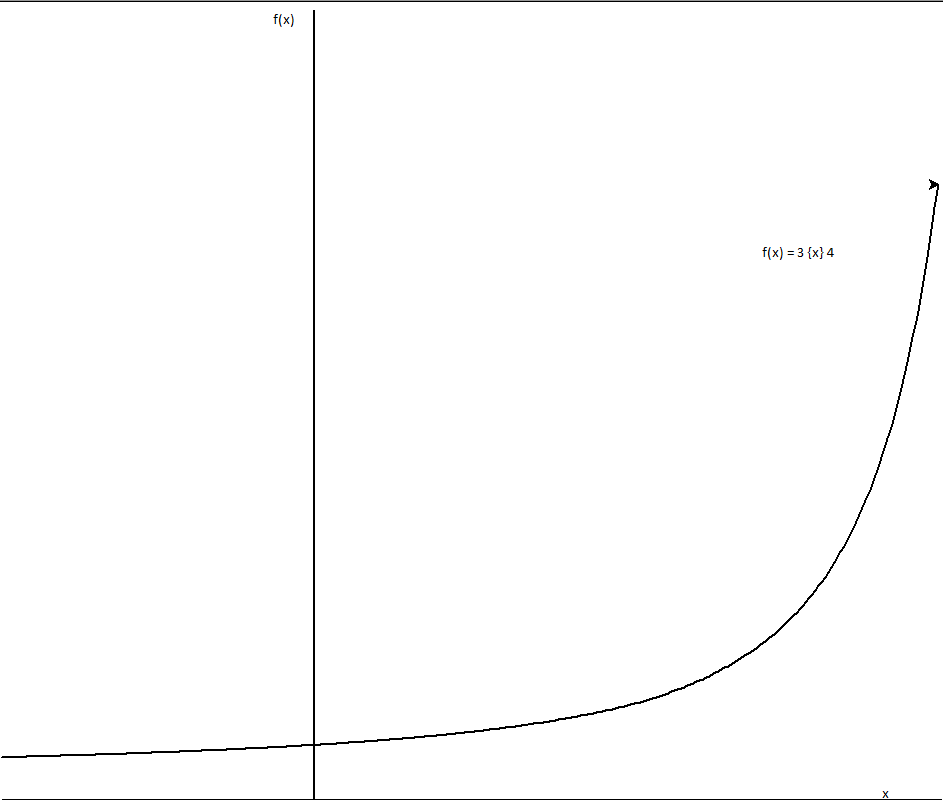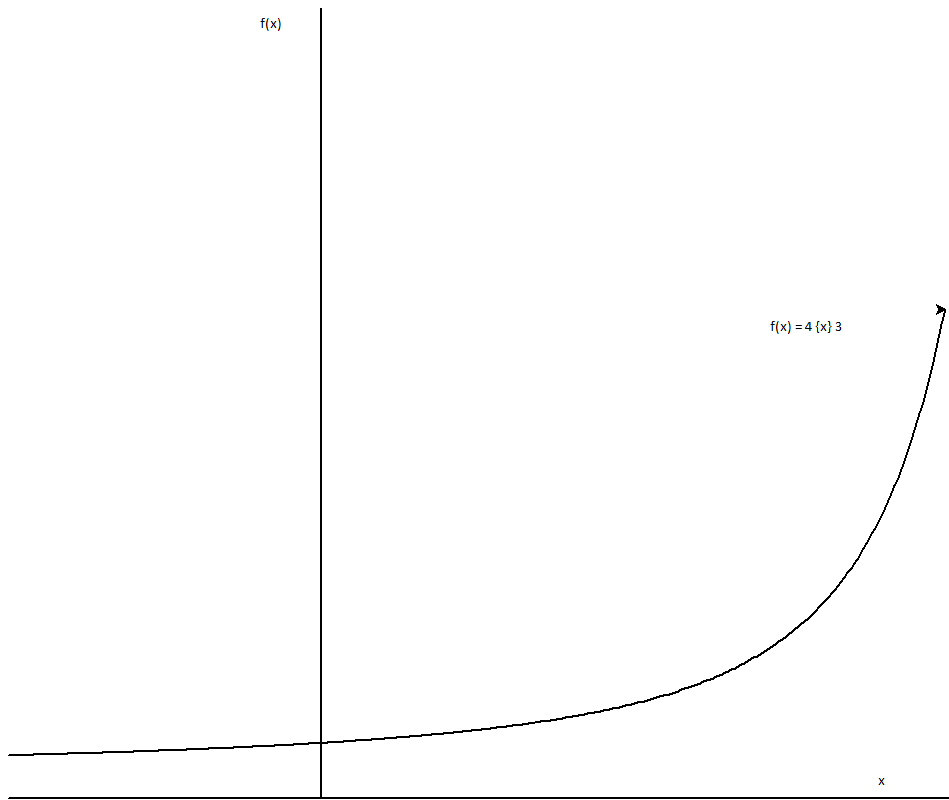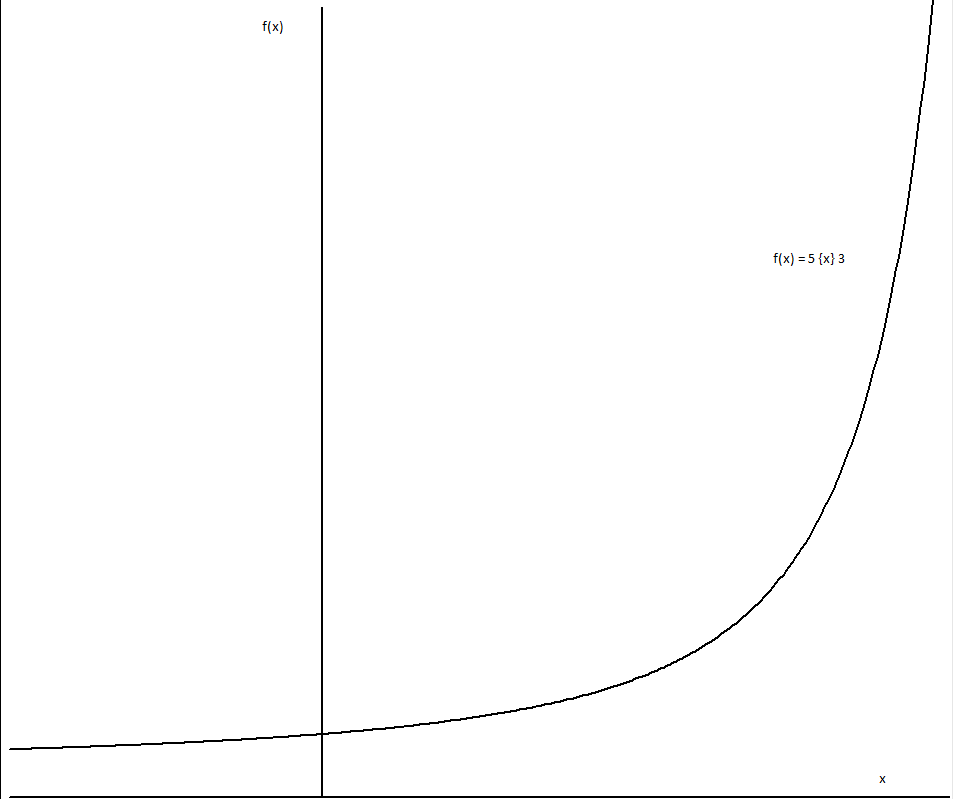• 0 Vote(s) - 0 Average
• 1
• 2
• 3
• 4
• 5
 Rational operators (a {t} b); a,b > e solvedJmsNxnLong Time FellowPosts: 291 Threads: 67 Joined: Dec 2010 06/08/2011, 11:47 PM (This post was last modified: 06/09/2011, 01:53 AM by JmsNxn.) (06/08/2011, 08:32 PM)sheldonison Wrote: So we have a definition for t=0..2 (addition, multiplication, exponentiation), for all values of a. Nice! So, now, I'm going to define the function f(b), which returns the base which has the fixed point of "b". , since e is the fixed point of b= , since 2 is the lower fixed point of b=sqrt(2) , since 3 is the upper fixed point of this base , since 4 is the upper fixed point of b=sqrt(2) , since 5 is the upper fixed point of this base I don't know how to calculate the base from the fixed point, but that's the function we need, and we would like the function to be analytic! This also explains why the approximation of using base eta pretty well, since the base we're going to use isn't going to be much smaller than eta, as b gets bigger or smaller than e. Now, we use this new function in place of eta, in James's equation. Here, f=f(b). - Sheldon Woah, I wonder what consequences this will have on the algebra. I guess which I guess isn't too drastic. But ,the question is, of course, does the following still hold : , so no it doesn't. That's not good, we want operators to be recursive. And I'm unsure if the inverse is still well defined, so I think we lose: where S(q) is the identity function. We may even lose the identity function altogether, this is really bad. We also lose: These are all too many valuable qualities that are lost when redefining semi-operators the way that you do. Sure it's analytic over , but it loses all its traits which make it an operator in the first place. I'm going to have to stick with the original definition of that isn't fully analytic. However, I am willing to concede the idea of changing from base eta to base root 2. That is to say if we define: This will give the time honoured result, and aesthetic necessity in my point of view, of: for all I like this also because it makes and potentially analytic over since 2 and 4 are fix points. I also propose writing Sheldon's analytic function is then: that's still very pretty though, that isn't piecewise over and potentially analytic.GottfriedUltimate FellowPosts: 766 Threads: 119 Joined: Aug 2007 06/11/2011, 02:33 PM Hi James, I do not really know, whether the following matches your input here; but screening through older discussions I just found an older post of Mike (I'd saved it by copying from google.groups). He observed the following and asked Henryk had answered with some proof of convergence and rate of convergence. I had an idea to reformulate this in a way using somehow "lower degree operators" than addition but could not make it better computable, so I didn't involve then further. If I get your approach right this can be used for such "lower order" operators? Say and for the h-fold iterated log( 3) then Mike's limit can be expressed where the operator-precedence is lower the more negative the index at the plus is (so we evaluate it from the left). First question: is this in fact an application of your "rational operator"? And if it is so, then second question: does this help to evaluate this to higher depth of iteration than we can do it when we try it just by log and exp alone (we can do it to iteration 4 or 5 at max I think) ? Gottfried cite: Quote:In article <20f2d3ea-1e87-45dc-86b2-a917f89e9370@i18g2000pro.googlegroups.com>, mike3 wrote: > Hi. > > I noticed this. > > log(3) ~ 1.098612288668109691395245237 > log(log(3^3)) ~ 1.192660116284808707569579569 > log(log(log(3^3^3))) ~ 1.220795907132767865324020633 > log(log(log(log(3^3^3^3)))) ~ 1.221729301870251716316203810 > (calculated indirectly via identity log(x^y) = y log(x).) > log(log(log(log(log(3^3^3^3^3))))) ~ 1.221729301870251827504003124 > (calculated indirectly via identity log(log(x^x^y)) = y log(x) + log > (log(x)).) > > It seems to be stabilizing on some weird value, around 1.2217293. > What is this? And we seem to run out of log identities here making > it infeasible to compute further approximations. > > Has this been examined before? Gottfried Helms, KasselJmsNxnLong Time FellowPosts: 291 Threads: 67 Joined: Dec 2010 06/12/2011, 07:55 PM (06/11/2011, 02:33 PM)Gottfried Wrote: Hi James, Yes this is right: I did a lot of investigation into this operator (well, to the best that I could). Quote:and for the h-fold iterated log( 3) then Mike's limit can be expressed where the operator-precedence is lower the more negative the index at the plus is (so we evaluate it from the left). First question: is this in fact an application of your "rational operator"? Well it appears to be. I'm floored, I'm terrible at finding applications. Quote:And if it is so, then second question: does this help to evaluate this to higher depth of iteration than we can do it when we try it just by log and exp alone (we can do it to iteration 4 or 5 at max I think) ? Well, not so far since the calculations involved in lower order operators rely on iterations of exp. However, I investigated in seeing if was analytic (which should help in calculations). But I only made it to the sixth or seventh derivative before I realized I wasn't going to recognize the pattern. The thread's here http://www.mymathforum.com/viewtopic.php?f=23&t=20993 . I assume it would be analytic, (the function looks analytic when graphed, if that's any argument). Also, I think that if is analytic, then is probably analytic, since it's basically the same function with just a faster convergence to y=x and a higher starting point at negative infinity.XorterFellowPosts: 92 Threads: 30 Joined: Aug 2016 08/21/2016, 06:56 PM (06/06/2011, 02:45 AM)JmsNxn Wrote: Well alas, logarithmic semi operators have paid off and have given a beautiful smooth curve over domain . This solution for rational operators is given by : Which extends the ackerman function to domain real (given the restrictions provided). the upper superfunction of is used (i.e: the cheta function). Logarithmic semi-operators contain infinite rings and infinite abelian groups. In so far as {t} and {t-1} always form a ring and {t-1} is always an abelian group (therefore any operator greater than {1} is not commutative and is not abelian). There is an identity function S(t), however its values occur below e and are therefore still unknown for operators less than {1} (except at negative integers where it is a variant of infinity (therefore difficult to play with) and at 0 where it is 0). Greater than {1} operators have identity 1. The difficulty is, if we use the lower superfunction of to define values less than e we get a hump in the middle of our transformation from . Therefore we have difficulty in defining an inverse for rational exponentiation. however, we still have a piecewise formula: therefore rational roots, the inverse of rational exponentiation is defined so long as and . rational division and rational subtraction is possible if and . Here are some graphs, I'm sorry about their poor quality but I'm rather new to pari-gp so I don't know how to draw graphs using it. I'm stuck using python right now. Nonetheless here are the graphs. the window for these ones is xmin = -1, xmax = 2, ymin = 0, ymax = 100If there's any transformation someone would like to see specifically, please just ask me. I wanted to do the transformation of as we slowly raise t, but the graph doesn't look too good since x > e. Some numerical values: (I know I'm not supposed to be able to calculate the second one, but that's the power of recursion) I'm very excited by this, I wonder if anyone has any questions comments? for more on rational operators in general, see the identities they follow on this thread http://math.eretrandre.org/tetrationforu...hp?tid=546 thanks, James PS: thanks go to Sheldon for the taylor series approximations of cheta and its inverse which allowed for the calculations. Hello, James! Hello, Everyone! I am really interested in that how you could make that beautiful graph. Could you tell me, please? Thank you very much. Xorter UnizoJmsNxnLong Time FellowPosts: 291 Threads: 67 Joined: Dec 2010 08/22/2016, 12:36 AM (This post was last modified: 08/22/2016, 12:41 AM by JmsNxn.) (08/21/2016, 06:56 PM)Xorter Wrote: Hello, James! Hello, Everyone! I am really interested in that how you could make that beautiful graph. Could you tell me, please? Thank you very much. Search for the cheta function on the forum and get its power series. Take define continuous solution which is analytic for t < 1 and t >1 with a singularity at t=1 oddly enough is analytic everywhere.XorterFellowPosts: 92 Threads: 30 Joined: Aug 2016 08/24/2016, 07:24 PM (08/22/2016, 12:36 AM)JmsNxn Wrote: (08/21/2016, 06:56 PM)Xorter Wrote: Hello, James! Hello, Everyone! I am really interested in that how you could make that beautiful graph. Could you tell me, please? Thank you very much. Search for the cheta function on the forum and get its power series. Take define continuous solution which is analytic for t < 1 and t >1 with a singularity at t=1 oddly enough is analytic everywhere. Thank you for the answer! Could you show me an example, too, please? E. g. How can I evaluate 3[0.5]3 or 3[1.5]3? Xorter UnizoXorterFellowPosts: 92 Threads: 30 Joined: Aug 2016 08/29/2016, 02:06 PM According to James' formula I could not tetrate. For example: 23 should be 2^^3 = 16, but according to the formula: 23 = f(2,3f(-2,2)) = f(2,3*1.869...) = 18.125... But why?Xorter UnizoJmsNxnLong Time FellowPosts: 291 Threads: 67 Joined: Dec 2010 09/01/2016, 06:47 PM (This post was last modified: 09/01/2016, 06:49 PM by JmsNxn.) (08/29/2016, 02:06 PM)Xorter Wrote: According to James' formula I could not tetrate. For example: 23 should be 2^^3 = 16, but according to the formula: 23 = f(2,3f(-2,2)) = f(2,3*1.869...) = 18.125... But why?The formula only works for in x [t] y The formula isn't really that valuable.tommy1729Ultimate FellowPosts: 1,370 Threads: 335 Joined: Feb 2009 09/02/2016, 01:50 AM (06/08/2011, 09:14 PM)bo198214 Wrote: (06/08/2011, 08:32 PM)sheldonison Wrote: So, now, I'm going to define the function f(b), which returns the base which has the fixed point of "b". , since e is the fixed point of b= , since 2 is the lower fixed point of b=sqrt(2) , since 3 is the upper fixed point of this base , since 4 is the upper fixed point of b=sqrt(2) , since 5 is the upper fixed point of this base I don't know how to calculate the base from the fixed point, but that's the function we need, and we would like the function to be analytic! Oh, Sheldon seems to be quite tired from all the calculation and discussion. Sheldon, give your self some time to rest! Your function isAbout those fixpoints and bases. Bo is correct ofcourse but i wanted to add how to find the other fixpoint. I end with a joke because i did NOT show which T is the correct one : it is the SMALLEST > 1 to be precise ... Analytic continuation is hard for a proof of that minimal property. The convex nature and fast growth of exp type functions is intuitive but also IMHO unconvincing / informal / weak. So no satisfying proof. Perhaps food for thought. ( certainly possible ! ) Anyway here it is ( and T = t ) X^1/x = y^1/y Ln(x)/ x = ln(y)/y Ln(x)/x = ln(T x)/ T x T ^ 1/T x^1/T = x T x = x^t X^(t - 1) = t Ofcourse new similar problemt1 ^ (1/(t1 - 1)) = t2 ^ (1/(t2 - 1)) Regards Tommy1729 The mastertommy1729Ultimate FellowPosts: 1,370 Threads: 335 Joined: Feb 2009 09/02/2016, 02:04 AM Oh dear e ^(T - 1) = T for T <> 0 gave me the idea of " fake fixpoint theory " in analogue to " fake function theory ". Apart from that funny/annoying thing , another reason is that this innocent little thing apparantly f* Up Some proof strategies for the desired Nice proof mentioned b4. Regards Tommy1729 « Next Oldest | Next Newest »

 Possibly Related Threads... Thread Author Replies Views Last Post Thoughts on hyper-operations of rational but non-integer orders? VSO 2 948 09/09/2019, 10:38 PM Last Post: tommy1729 Hyper operators in computability theory JmsNxn 5 4,751 02/15/2017, 10:07 PM Last Post: MphLee Recursive formula generating bounded hyper-operators JmsNxn 0 1,722 01/17/2017, 05:10 AM Last Post: JmsNxn holomorphic binary operators over naturals; generalized hyper operators JmsNxn 15 17,922 08/22/2016, 12:19 AM Last Post: JmsNxn The bounded analytic semiHyper-operators JmsNxn 2 3,982 05/27/2016, 04:03 AM Last Post: JmsNxn Bounded Analytic Hyper operators JmsNxn 25 22,547 04/01/2015, 06:09 PM Last Post: MphLee Incredible reduction for Hyper operators JmsNxn 0 2,464 02/13/2014, 06:20 PM Last Post: JmsNxn interpolating the hyper operators JmsNxn 3 5,551 06/07/2013, 09:03 PM Last Post: JmsNxn Number theory and hyper operators JmsNxn 7 8,438 05/29/2013, 09:24 PM Last Post: MphLee Number theoretic formula for hyper operators (-oo, 2] at prime numbers JmsNxn 2 4,372 07/17/2012, 02:12 AM Last Post: JmsNxn

Users browsing this thread: 1 Guest(s)